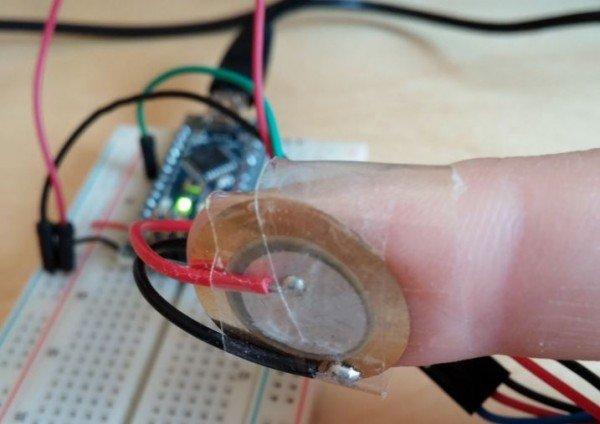# Measuring Heart Rate With A Piezo

While trying to create a circuit that detects whether water is flowing through a pipe by measuring the vibration with a piezoelectric sensor, just to see what happens I taped the sensor around my finger and – to my surprise – got values that were a very noise-free representation of my heart rate!

This is even easier than using LEDs as it only requires a piezoelectric sensor and an Arduino. (and a piece of tape)
The sensor I used is a DFRobot Piezo Disc Vibration Sensor Module.void loop() {
int avg = 0;
for(int i=0;i<64;i++){
}
Serial.println(avg/64,DEC);
delay(5);
}
 123456789101112 void setup() {  Serial.begin(57600);}void loop() {  int avg = 0;  for(int i=0;i<64;i++){    avg+=analogRead(A2);  }  Serial.println(avg/64,DEC);  delay(5);}

When defining an arbitrary threshold (e.g. half of the maximum measured value), the rising edge of the signal will pass the threshold once per heartbeat, making measuring it as simple as measuring the time between two successive beats. For less jitter, I chose to calculate the heart rate using the average of the last 16 time differences between the beats.

Here’s a quick and dirty code that calculates the heart rate and outputs the average heart rate over the last 16 beats at every beat:

int threshold = 60;
int oldvalue = 0;
int newvalue = 0;
unsigned long oldmillis = 0;
unsigned long newmillis = 0;
int cnt = 0;
int timings;
void setup() {
Serial.begin(57600);}
void loop() {  oldvalue = newvalue;
newvalue = 0;  for(int i=0; i<64; i++){ // Average over 16 measurements
newvalue = newvalue/64;  // find triggering edge
if(oldvalue<threshold && newvalue>=threshold){
oldmillis = newmillis;
newmillis = millis();
// fill in the current time difference in ringbuffer
timings[cnt%16]= (int)(newmillis-oldmillis);
int totalmillis = 0;  }

#### This Post / Project can also be found using search terms:

• arduino measuring heart rate
• finger print sensor vibration
• heart rate piezo
• heart rate piezo code arduino